# Pre-Algebra : Associative Property of Multiplication

## Example Questions

### Example Question #1 : Associative Property Of Multiplication

If the below expression is written using the Associative Property of Multiplication, what are the values ofand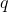?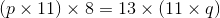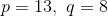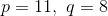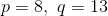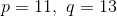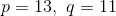Explanation:

The rule for Associative Property of Multiplication is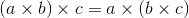Using this rule, we can determine that for the given expression,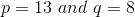### Example Question #2 : Associative Property Of Multiplication

Which set of equations illustrates the Associative property of Multiplication?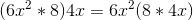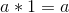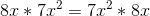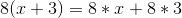Explanation:is the distributive property. Multiply each term in the parenthesis by the outside term.is the Commutative property of Multiplication. The order of two like terms being multiplied does not change the product.is the Multiplicative Identity property. Anything multiplied by 1 does not change.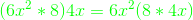is Associative property of Multiplication. The grouping of terms does not change their product as long as all terms remain in the equation. Try the green equation without the variables to see for yourself.

### Example Question #21 : Identities And Properties

Which of the following best represents the associative property of multiplication?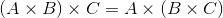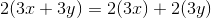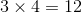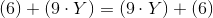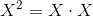Explanation:

The property states that changing the order of multiplying any two numbers will not change the final answer.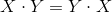The answeris the best choice.

### Example Question #1 : Associative Property Of Multiplication

Which of the following displays the associative property of multiplication?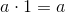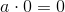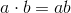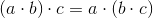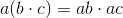Explanation:

The associative property of multiplication states that you can multiply numbers together in any order, and the answer will not change.  Therefore,displays the associative property of multiplication since the two sides are equal no matter which set of parentheses you solve first.

### All Pre-Algebra Resources